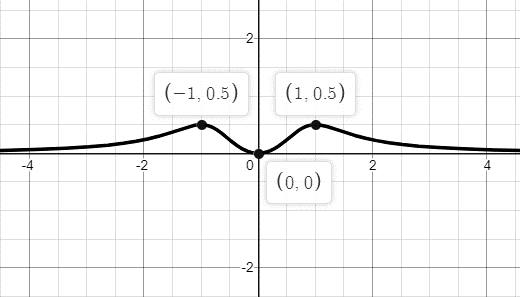# Whether the given functionis even, odd, or neither.### Single Variable Calculus: Concepts...

4th Edition
James Stewart
Publisher: Cengage Learning
ISBN: 9781337687805### Single Variable Calculus: Concepts...

4th Edition
James Stewart
Publisher: Cengage Learning
ISBN: 9781337687805

#### Solutions

Chapter 1.1, Problem 68E
To determine

## To find: Whether the given functionis even, odd, or neither.

Expert Solution

The given function is an even function.

### Explanation of Solution

Given information:

The given function is,

f(x)=x2x4+1

Concept used:

The function is said to be odd function if it satisfies f(x)=f(x) for all values of x and the function is said to be even function if it satisfies f(x)=f(x) for all values of x .

The given function is,

f(x)=x2x4+1

Calculate the value of f(x) as,

f(x)=(x)2(x)4+1f(x)=x2x4+1f(x)=f(x)

The given function satisfies the condition of an even function. Hence, the given function is an even function.

Consider the graph of the given function as follows,### Have a homework question?

Subscribe to bartleby learn! Ask subject matter experts 30 homework questions each month. Plus, you’ll have access to millions of step-by-step textbook answers!